• 针对卫星姿态估计的非线性、非高斯特性, 提出一种粒子滤波预测滤波相结合的估计方法, 在无角速率测 量时, 首先利用预测方法在线估计系统模型误差和姿态角速度, 再通过改进的规则化粒子滤波器估计姿态四元数. ...
• 粒子滤波实现刀具寿命预测（附python代码） 背景介绍 刀具失效是加工过程中的主要问题，通过多特征融合方法实现刀具磨损量预测后建立了刀具的健康指标。接下来就是利用得到的健康指标对刀具的剩余寿命进行预测粒子...
粒子滤波实现刀具寿命预测（附python代码）
背景介绍
刀具失效是加工过程中的主要问题，通过多特征融合方法实现刀具磨损量预测后建立了刀具的健康指标。接下来就是利用得到的健康指标对刀具的剩余寿命进行预测。粒子滤波则是一种常用的方法。
关于粒子滤波的理论知识参见粒子滤波理论。
本文主要讲解通过python简单实现基于粒子滤波的刀具寿命预测思路以及简要的代码。
粒子滤波的主要流程
1、建立刀具退化模型。结合刀具退化规律，一般情况下我们使用双指数模型。即：
$\ z_k$=$\ ae^{bk}$+$\ ce^{dk}$
2、初始化参数与粒子集初始化。$\ k=0$时根据先验生成M个$\ x_0$采样粒子，即：{$x_0^{(i)}$}$\ _{i=1}^M$。
3、 进行粒子滤波。对于$\ k=1,2,3...t$
（1）重要性采样。生成采样粒子{$x_k^{(i)}$}$\ _{i=1}^M$，计算粒子权值$w_k$，并归一化；
（2）重采样。重采样后的粒子集为{$x_k^{(i)},1/M$}；
（3）输出。计算$k$时刻的状态估计值，$\widehat{x}=$$\sum_{i=1}^{M} x_k^{(i)}w_k^{(i)}$。
4、剩余寿命预测。将粒子集参数带入刀具退化模型完成寿命预测。
（1）将M个粒子带入退化模型得到刀具当前状态后的退化趋势；
（2）设定阈值，当磨损状态超过阈值后即判断刀具失效，通过剩余寿命方程$\ L_t=inf$⁡{$\ l_t:Y(t+l_t)≥D,t>0$}得到M个粒子的刀具剩余寿命。
（3）取M个粒子得到的剩余寿命的均值作为最终的刀具剩余寿命。粒子滤波
居中并且带尺寸的图片:退化模型
相关代码
初始化

##initial value of model parameters

a = random.uniform(0.0147, 0.01475)
b = random.uniform(0.0556, 0.0558)
c = random.uniform(0.250, 0.255)
d = random.uniform(0.0035, 0.0037)
X0 = np.array([[a], [b], [c], [d]])

##Parameters for Particle Filter
M = 100   #粒子个数
p = 4      #参数个数
Xparam = np.zeros([p, N])
Xparam[:,0] = X0[:,0]

##Process Noise and Measurement Noise
var_a = 0.000001
var_b = 0.01
var_c = 0.1
var_d = 0.0001
sd_z = 1E-4
Q = sd_z * np.diag([var_a, var_b, var_c, var_d])
F = np.eye(p)
R = 0.001

##Monte Carlo Simulation 粒子集初始化
Xm = np.zeros([p, M, N])
for i in range(0, M):
dx1 = X0 + np.dot(sl.sqrtm(Q), np.random.randn(p, 1))
Xm[:, i, 0] = dx1[:,0]


粒子滤波
 #Particle Filtering
for k in range(2, N+1):
#state transition equations
for i in range(0, M):
Xm[0,i,k-1] = Xm[0,i,k-2] + np.sqrt(var_a*sd_z)*np.random.randn()
Xm[1,i,k-1] = Xm[1,i,k-2] + np.sqrt(var_b*sd_z)*np.random.randn()
Xm[2,i,k-1] = Xm[2,i,k-2] + np.sqrt(var_c*sd_z)*np.random.randn()
Xm[3,i,k-1] = Xm[3,i,k-2] + np.sqrt(var_d*sd_z)*np.random.randn()

#Weighing of particles
for i in range(0, M-1):
Zm[0,i,k-1] = Xm[0,i,k-1] * np.exp(Xm[1,i,k-1]*k) + Xm[2,i,k-1] * np.exp(Xm[3,i,k-1]*k)
W[k-1,i] = np.exp(-(Zmeasured[0,k-1]-Zm[0,i,k-1])**2/2/R) +1e-99

#Resampling based on weights
W[k-1,:] = W[k-1,:] / np.sum(W[k-1,:])                #权值归一化
dx3 = []
for i in range(1, M+1):
dx3.append(i)
dx3 = np.array([dx3])
outIndex = residualR(dx3, np.array([W[k-1,:]]).T)     #随机重采样
Xm[:,:,k-1] = Xm[:,outIndex[:], k-1]                     #得到新的样本集
#value of particles
for i in range(M):
Ae[0, i, k-1] = Xm[0, i, k-1]
Be[0, i, k-1] = Xm[1, i, k-1]
Ce[0, i, k-1] = Xm[2, i, k-1]
De[0, i, k-1] = Xm[3, i, k-1]
Zpf[0,i,k-1] = Ae[0, i, k-1] * np.exp(Be[0, i, k-1] * k/1000) + Ce[0, i, k-1]*np.exp(De[0, i, k-1]*k/1000)+sd_z * np.random.randn()

剩余寿命预测
 Zf1 = np.zeros([1, M, noOfCycles])
Xf1 = np.zeros([1, M, noOfCycles])
for i in range(M):
for k in range(int(start), noOfCycles):
Zf1[0,i, k - start] = Ae[0, i, start - 1] * np.exp(Be[0, i, start - 1] * k/1000) + Ce[0, i, start - 1]*np.exp(De[0, i, start-1]*k/1000)+sd_z * np.random.randn()
Xf1[0,i, k - start] = k
#RUL
if Zf1[0, i, k - start] >= threshold_capacity:
failure_index = k



展开全文python
• 粒子滤波初探 对于线性、高斯分布的运动模型，有卡尔曼滤波，那么对于非线性、非高斯的运动模型，粒子滤波器发挥出其优越性，主体思想是通过大量试验，对正确的试验状态予以适当的权重分配，从而实现根据权重增加的...
粒子滤波初探

对于线性、高斯分布的运动模型，有卡尔曼滤波，那么对于非线性、非高斯的运动模型，粒子滤波器发挥出其优越性，主体思想是通过大量试验，对正确的试验状态予以适当的权重分配，从而实现根据权重增加的方向实现跟踪。

本文与其他描述粒子滤波的博文不同，先以例子引入，让初步了解的人对粒子滤波有一个大致认识。

以视频的目标跟踪为例，解说一下这个粒子滤波跟踪流程。

一、t-1时刻下：假定我们有这么一个视频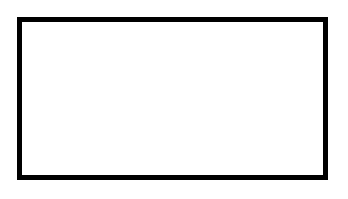二、我们来初始化这个粒子滤波器

使上面的屏幕布满粒子
初始化每个粒子的权重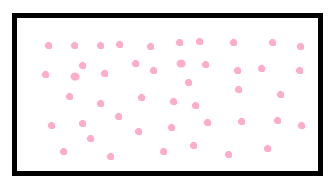三、如果视频中有个人，而且被我们框定作为目标

在利用重要性采样计算粒子权重之前，要记住这时候的粒子还是布满整个屏幕的，而且权重一样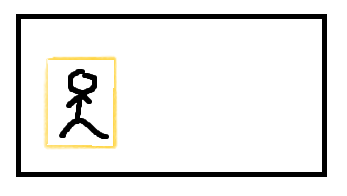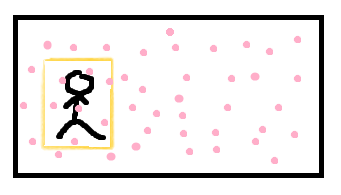四、更新权重

这时候，由于我们有了目标，就可以去计算粒子的权重了（我们暂时不去了解如何计算新的权重，反正粒子附近的图像与我们框定的图像内容越相似，其得到的更新权重就越高【想要了解的可看下一篇《粒子滤波初探（二）利用粒子滤波实现视频目标跟踪工程实战》的步骤四】）
下图中，颜色越鲜艳的代表该粒子权重越大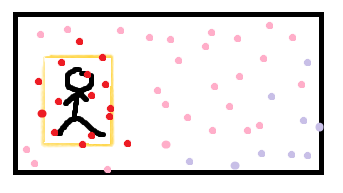五、重采样

接下来，很自然的想到，舍弃权重较小的粒子，但是为了维持粒子数不变，自然是要增加一些粒子，这就是重采样
于是有了下图
粒子群集中到我们框定的目标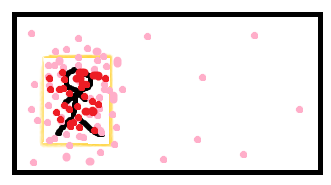六、然后，根据现在的权重分配及给定的状态转移矩阵，预测（生成）出下一时刻即t时刻粒子群的位置

假定预测的结果是这个样子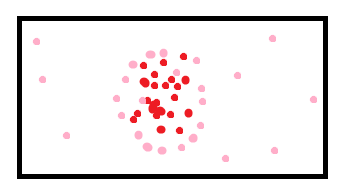以上都是t-1时刻下操作

七、进入t时刻（我以视频边框*2表示）

假定我们的人已经走到这个位置
下面4张图，上面两张是t-1时刻下的，下面两张是t时刻的
左下是我们的行人移动的位置
右下是我们的行人和t-1时刻预测出来的t时刻的粒子位置
从右下图可见，我们的预测貌似偏了一些，没关系，下一步将重新计算粒子的权重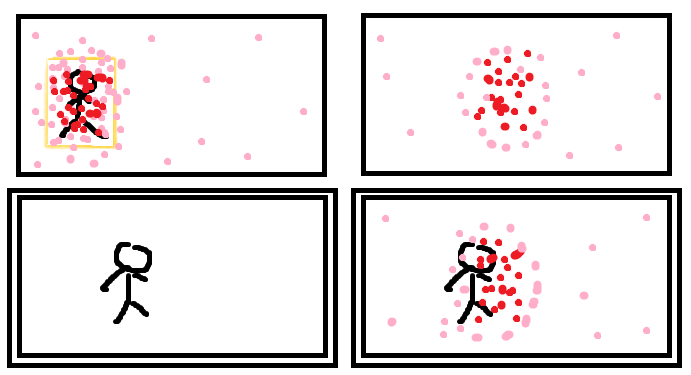八、更新权重

跟第四步一样，我们以t-1时刻下我们手动标注的橙色框为目标，重新更新我们粒子的权重
粒子还是那些粒子，只不过权重发生变化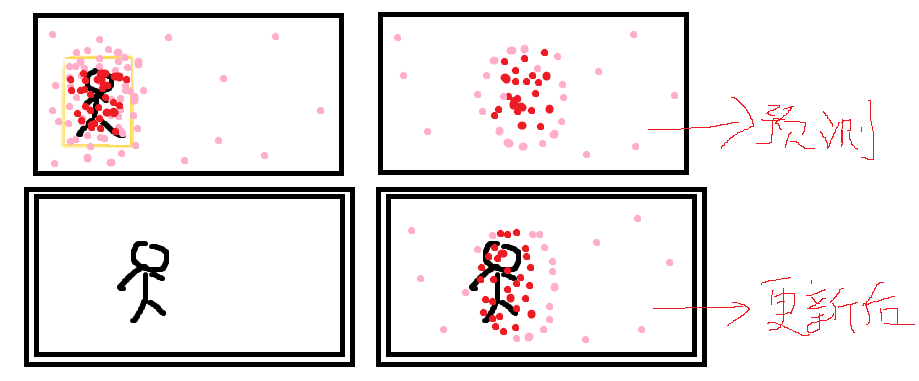九、重采样&&提取位置

重采样：去除权重低的粒子，复制权重高的粒子
根据重采样后的粒子分布，可以获取大致的目标位置，也就是我们跟踪的成果，如右下图蓝框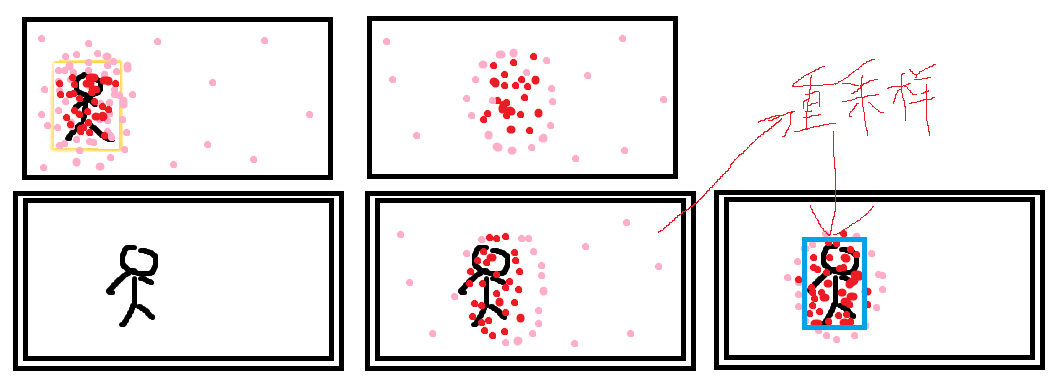十、更新目标

这里已经是t时刻的末端：
我们以此刻蓝框的内容作为我们的目标，以便t+1时刻用以更新粒子权重
我们以粒子群从t-1时刻到t时刻重采样后（即从橙色框移动到蓝色框的运动方向），作为粒子群下一次运动方向的依据，预测（生成）出t+1时刻粒子群的位置，接下来的步骤同第六步
到这里，t时刻结束，进入t+1时刻
到这里，一共十步，大致把这个粒子滤波的目标跟踪流程理了一遍。

下一篇：粒子滤波初探（二）利用粒子滤波实现视频目标跟踪工程实战 ，有可运行代码，是个人根据几位先驱做作出的努力翻译到opencv2.49下的，并做出了一些修正，希望各位指出不足和作出修正

参考资料：

https://blog.csdn.net/guoyunlei/article/details/78183530

https://blog.csdn.net/gwplovekimi/article/details/80359512

https://blog.csdn.net/yang_xian521/article/details/6928131

http://www.cnblogs.com/yangyangcv/archive/2010/05/23/1742263.html
展开全文• 结合灰色预测模型和粒子滤波, 提出一种新的视觉目标跟踪算法. 由于粒子滤波未考虑先验信息对建议分 布产生的指导作用, 不能很好地逼近后验概率分布, 对此, 采用历史状态估计序列作为先验信息, 建立该序列的灰色...
• 利用卡尔曼嵌入粒子滤波的方法对粒子滤波预测的状态值进行二次预测，并且利用二次采样技术增强粒子的丰富度，从而在一定程度上消除背景噪声的影响。同时为了满足卡尔曼滤波对线性运动的要求以及消除背景快速变化对...
• 粒子滤波在单目标跟踪多目标跟踪电池寿命预测中的应用-粒子滤波原理及应用仿真.doc 本帖最后由 huangxu_love 于 2013-7-26 12:50 编辑 推荐一本学习粒子滤波原理的好资料《粒子滤波原理及应用仿真》，本手册...matlab
•研究论文
• ## 粒子滤波代码

千次下载 热门讨论 2014-11-15 12:00:39
压缩包中有三个粒子滤波的演示程序，一个滤波，一个目标跟踪，一个机器人定位。关于效果，大家可以先看看http://blog.csdn.net/heyijia0327/article/details/41142679。再决定是否下载。
• 基于粒子滤波的故障预测时间方法，具有实际的预测应用程序研究论文
• ## 粒子滤波

千次阅读 2019-11-27 09:51:00
2. 粒子滤波 2.1 概念 2.2 粒子滤波算法原理 2.3 局限性 3. 代码实现 1. 状态空间模型 状态空间模型是动态时域模型，以隐含着的时间为自变量。状态空间模型在经济时间序列分析中的应用正在迅速增加。其中...
目录

1. 状态空间模型

2. 粒子滤波

2.1 概念

2.2 粒子滤波算法原理

2.3 局限性

3. 代码实现

1. 状态空间模型

状态空间模型是动态时域模型，以隐含着的时间为自变量。状态空间模型在经济时间序列分析中的应用正在迅速增加。其中应用较为普遍的状态空间模型是由Akaike提出并由Mehra进一步发展而成的典型相关(canonical correlation)方法。

状态空间模型的假设条件是动态系统符合马尔科夫特性，即给定系统的现在状态，则系统的将来与其过去独立。

状态空间模型具有如下特点：

1、状态空间模型不仅能反映系统内部状态，而且能揭示系统内部状态与外部的输入和输出变量的联系。
2、状态空间模型将多个变量时间序列处理为向量时间序列，这种从变量到向量的转变更适合解决多输入输出变量情况下的建模问题。
3、状态空间模型能够用现在和过去的最小心信息形式描述系统的状态，因此，它不需要大量的历史数据资料，既省时又省力。
基于状态空间模型的时间序列预测的优点：

优点一：状态空间模型是一种结构模型，基于状态空间分解模型的时间序列预测，便于分析者利用存在的统计理论对模型进行统计检验。
优点二：状态空间模型求解算法的核心是Kalman滤波，Kalman滤波是在时刻t基于所有可得到的信息计算状态向量的最理想的递推过程。当扰动项和初始状态向量服从正态分布时，Kalman滤波能够通过预测误差分解计算似然函数，从而可以对模型中的所有未知参数进行估计，并且当新的观测值一旦得到，就可以利用Kalman滤波连续地修正状态向量的估计。
2. 粒子滤波

2.1 概念

学习文章链接：

（1）https://blog.csdn.net/setella/article/details/82912604

（2）https://blog.csdn.net/guo1988kui/article/details/82778293

粒子滤波技术在非线性、非高斯系统表现出来的优越性，决定了它的应用范围非常广泛。另外，粒子滤波器的多模态处理能力，也是它应用广泛的原因之一。国际上，粒子滤波已被应用于各个领域。在经济学领域，它被应用在经济数据预测；在军事领域已经被应用于雷达跟踪空中飞行物，空对空、空对地的被动式跟踪；在交通管制领域它被应用在对车或人视频监控；它还用于机器人的全局定位。

粒子滤波的缺点：

虽然粒子滤波算法可以作为解决SLAM问题的有效手段，但是该算法仍然存在着一些问题。其中最主要的问题是需要用大量的样本数量才能很好地近似系统的后验概率密度。机器人面临的环境越复杂，描述后验概率分布所需要的样本数量就越多，算法的复杂度就越高。因此，能够有效地减少样本数量的自适应采样策略是该算法的重点。另外，重采样阶段会造成样本有效性和多样性的损失，导致样本贫化现象。如何保持粒子的有效性和多样性，克服样本贫化，也是该算法研究重点。

2.2 粒子滤波算法原理

粒子滤波是采用蒙特卡洛方法求解贝叶斯估计中的最优估计，并利用重要性采样方法在状态空间上得到一组不断更新的随机样本（称为粒子），这一组粒子对应着一组权值。通过不停的按照权值大小来更新系统中的粒子并更新权值，来逼近需要估计的系统状态。算法步骤主要分为如下几步：

（1）粒子初始化：由于对系统状态未知，所以我们认为粒子在系统的状态空间中均匀分布，初始化生成粒子。然后将所有采样输入状态转移方程，得到预测粒子。

（2）粒子预测阶段：粒子滤波根据上一时刻确定的后验概率分布，通过随机采样的方法对每一个粒子的值进行更新，再将更新过的粒子输入状态转移方程，得到一组预测值，通过这组预测值的加权组合得到该时刻的预测结果。

（3）权值更新阶段：在获取一组粒子产生的预测值后，对比该时刻真实的观测值，通过观测方程对预测值进行评价。即第i个粒子输入观测方程后能得到真实观测值的概率，令这个概率为该粒子的权重，越可能获得真实观测值的粒子对应的权重就越高，也就代表该粒子越符合真实的概率分布。

（4）粒子重采样：为了避免粒子退化的现象，在算法迭代步骤中，需要去除权值较低的粒子，对权值较高的粒子进行复制，使得粒子的分布位置更逼近真实的解。在下一次的滤波过程中，使用重采样之后的粒子，使得每一轮滤波都让粒子更符合真实状态的概率分布，再将粒子带入状态转移方程中进行预测，从而使得预测的结果更准确。
如图4-3所示，如此反复迭代上述几个阶段，将得到状态转移方程的最优估计，即我们需要的预测值。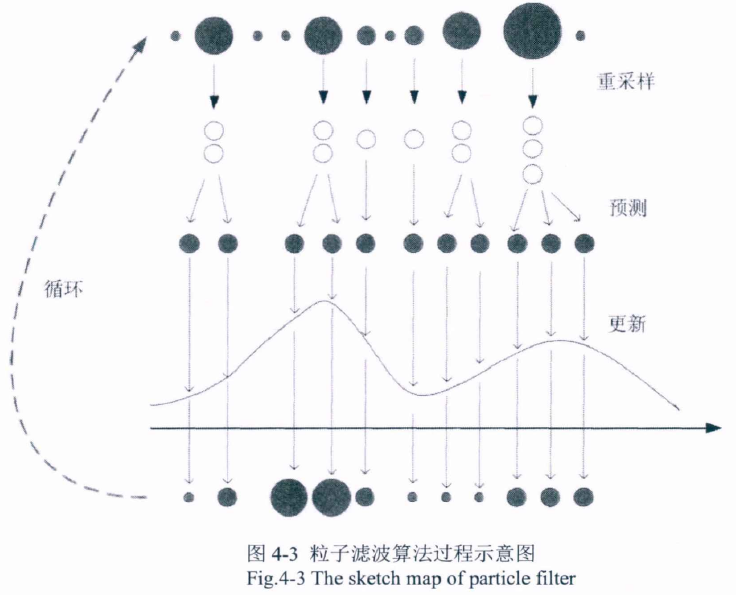2.3 局限性

对于粒子滤波来说，预测过程的实现必须依赖于系统指定的状态转移方程和观测方程，但是现金流无法建立估计式模型。若随机初始化粒子，则无法实现预测，如下图所示：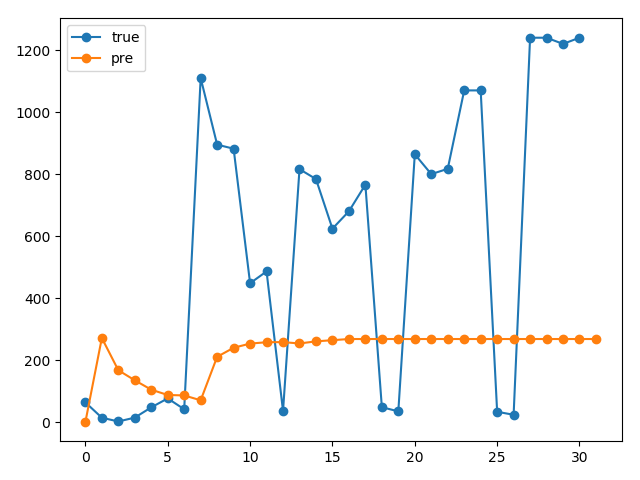因此，使用粒子滤波，必须提供估计式，或者与其他算法结合使用：

xk与xk-1、xk与yk的关系，建立固定方程式；
与神经网络、SVM等算法结合，使用算法的预测结果作为粒子，不同参数的NN预测结果 = 不同的粒子。
与神经网络、SVM等算法结合本质：集成算法，基学习器是NN，通过粒子权值更新来求各个NN的权重

状态转移方程 = 神经网络预测过程
预测过程：神经网络预测，作为粒子预测
更新过程：使用观测值，调整各个NN的权重
3. 代码实现

import pandas as pd
import numpy as np
import math
import functools
import matplotlib.pyplot as plt

x = 0.1   # initial actual state
x_N = 1   # 系统过程噪声的协方差(由于是一维的，这里就是方差)
x_R = 1   # 测量的协方差
T = 75    #  共进行75次
N = 100   # 粒子数，越大效果越好，计算量也越大

# initilize our initial, prior particle distribution as a gaussian around the true initial value

V = 2               # 初始分布的方差
x_P = np.zeros(N)   # 粒子

# 1.用一个高斯分布随机的产生初始的粒子
for i in range(N):
x_P[i] = x + np.sqrt(V) * np.random.randn()

z_out = [x ** 2 / 20 + np.sqrt(x_R) ** np.random.randn()]  # 实际测量值
x_out = [x]     # the actual output vector for measurement values.测量值的实际输出矢量
x_est = [x]     # time by time output of the particle filters estimate 粒子滤波估计的逐时输出
x_est_out = [x]   # the vector of particle filter estimates. 粒子滤波估计的向量

# 重采样
def hinstc(listname, n):
ran_w = np.random.rand(n)
dd = []
for i in ran_w:
j = 0
while i > listname[j]:
if i < listname[j + 1]:
break
else:
j += 1
dd.append(j + 1)
return dd

x_P_update = np.zeros(N)
z_update = np.zeros(N)
P_w = np.zeros(N)
for t in range(T):
x = 0.5 * x + 25 * x / (1 + x ** 2) + 8 * np.cos(1.2 * (t - 1)) + np.sqrt(x_N) * np.random.randn()
z = x ** 2 / 20 + np.sqrt(x_R) * np.random.randn()

# 2.权值计算
for i in range(N):
x_P_update[i] = 0.5 * x_P[i] + 25 * x_P[i] / (1 + x_P[i] ** 2) + 8 * np.cos(1.2 * (t - 1)) + np.sqrt(x_N) * np.random.randn()
# 计算采样粒子的值，为后面根据似然去计算权重做铺垫
z_update[i] = x_P_update[i]** 2 / 20
# 对每个粒子计算其权重，这里假设量测噪声是高斯分布。所以w = p(y | x) 对应下面的计算公式
P_w[i] = (1 / np.sqrt(2 * np.pi * x_R)) * np.exp(-(z - z_update[i]) ** 2 / (2 * x_R))

# 归一化.
P_w = P_w / sum(P_w)

# 3.重采样 Resampling
cum = np.random.rand(N)
for j in range(N):
# reduce 累加
cum[j] = functools.reduce(lambda x, y: x + y, P_w[:j + 1])
dd = hinstc(cum, N)
for j in range(N):
x_P[j] = x_P_update[dd[j]]

# 状态估计，重采样以后，每个粒子的权重都变成了1 / N
x_est = np.mean(x_P)

# Save data in arrays for later plotting
x_out.append(x)  # 真实值
z_out.append(z)
x_est_out.append(x_est)  # 预测值

plt.figure()
plt.plot(x_out, label='true')
plt.plot(x_est_out, label='pre')
plt.legend()
plt.show()


结果如下：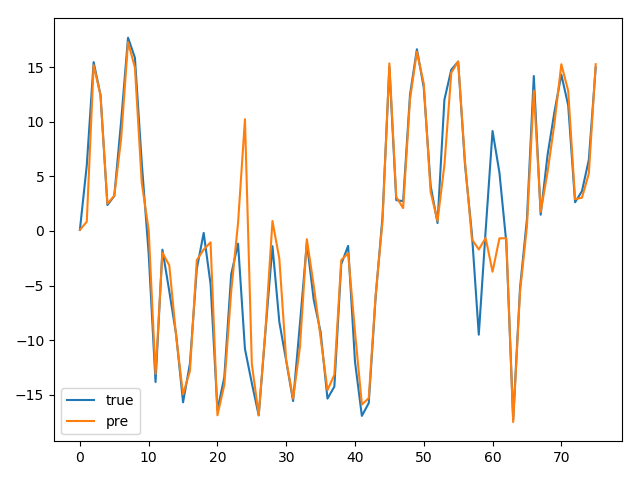参考：https://blog.csdn.net/heyijia0327/article/details/41142679

展开全文• 粒子滤波算法源于蒙特卡洛思想，即以某事件出现的频率来指代该事件的概率。在粒子滤波过程中，X(t)实际上是通过对大量粒子的状态进行处理得到的。粒子滤波的5个步骤：1)初始状态：用大量粒子模拟X(t)，粒子在空间内...
粒子滤波算法源于蒙特卡洛思想，即以某事件出现的频率来指代该事件的概率。在粒子滤波过程中，X(t)实际上是通过对大量粒子的状态进行处理得到的。粒子滤波的5个步骤：1)初始状态：用大量粒子模拟X(t)，粒子在空间内均匀分布；2)预测阶段：根据状态转移方程，每一个粒子得到一个预测粒子；3)校正阶段：对预测粒子进行评价，越接近于真实状态的粒子，其权重越大；4)重采样：根据粒子权重对粒子进行筛选，筛选过程中，既要大量保留权重大的粒子，又要有一小部分权重小的粒子；5)滤波：将重采样后的粒子带入状态转移方程得到新的预测粒子，即步骤2。%在二维空间,假设运动物体的一组(非线性)运动位置、速度、加速度数据,用粒子滤波方法进行处理%实验室的博客% 参数设置N = 100; %粒子总数Q =5; %过程噪声R =5; %测量噪声T =10; %测量时间theta =pi/T; %旋转角度distance =80/T; %每次走的距离WorldSize =100; %世界大小X = zeros(2,T); %存储系统状态Z = zeros(2,T); %存储系统的观测状态P = zeros(2,N); %建立粒子群PCenter = zeros(2, T); %所有粒子的中心位置w = zeros(N,1); %每个粒子的权重err =zeros(1,T); %误差X(:, 1) = [50;20]; %初始系统状态Z(:, 1) = [50; 20] + wgn(2, 1,10*log10(R)); %初始系统的观测状态%初始化粒子群for i = 1 : NP(:, i) =[WorldSize*rand; WorldSize*rand];dist =norm(P(:, i)-Z(:,1)); %与测量位置相差的距离w(i) = (1 /sqrt(R) / sqrt(2 * pi)) * exp(-(dist)^2 / 2 /R); %求权重endPCenter(:, 1) = sum(P, 2) /N; %所有粒子的几何中心位置%%err(1) = norm(X(:, 1) - PCenter(:,1)); %粒子几何中心与系统真实状态的误差figure(1);set(gca,'FontSize',12);hold onplot(X(1, 1), X(2, 1), 'r.','markersize',30) %系统状态位置axis([0 100 0 100]);plot(P(1, :), P(2, :), 'k.','markersize',5); %各个粒子位置plot(PCenter(1, 1), PCenter(2, 1), 'b.', 'markersize',25);%所有粒子的中心位置legend('True State', 'Particles', 'The Center of Particles');title('Initial State');hold off%%%开始运动for k = 2 : T%模拟一个弧线运动的状态X(:, k) =X(:, k-1) + distance * [(-cos(k * theta)); sin(k * theta)] + wgn(2,1,10*log10(Q)); %状态方程Z(:, k) =X(:, k) + wgn(2, 1,10*log10(R)); %观测方程 %粒子滤波%预测for i = 1 :NP(:, i) = P(:, i) + distance * [-cos(k * theta); sin(k * theta)] +wgn(2, 1, 10*log10(Q));dist = norm(P(:, i)-Z(:,k)); %与测量位置相差的距离w(i) = (1 / sqrt(R) / sqrt(2 * pi)) * exp(-(dist)^2 / 2 /R); %求权重end%归一化权重wsum =sum(w);for i = 1 :Nw(i) = w(i) / wsum;end%重采样(更新)for i = 1 :Nwmax = 2 * max(w) * rand; %另一种重采样规则index = randi(N, 1);while(wmax > w(index))wmax = wmax - w(index);index = index + 1;if index > Nindex = 1;end endP(:, i) = P(:,index); %得到新粒子endPCenter(:,k) = sum(P, 2) /N; %所有粒子的中心位置%计算误差err(k) =norm(X(:, k) - PCenter(:,k)); %粒子几何中心与系统真实状态的误差figure(2);set(gca,'FontSize',12);clf;holdonplot(X(1,k), X(2, k), 'r.', 'markersize',50); %系统状态位置axis([0 1000 100]);plot(P(1,:), P(2, :), 'k.','markersize',5); %各个粒子位置plot(PCenter(1, k), PCenter(2, k), 'b.', 'markersize',25);%所有粒子的中心位置legend('TrueState', 'Particle', 'The Center of Particles');holdoffpause(0.1);end%%figure(3);set(gca,'FontSize',12);plot(X(1,:), X(2,:), 'r', Z(1,:), Z(2,:), 'g', PCenter(1,:),PCenter(2,:), 'b-');axis([0 100 0 100]);legend('True State', 'Measurement', 'Particle Filter');xlabel('x', 'FontSize', 20); ylabel('y', 'FontSize', 20);%%figure(4);set(gca,'FontSize',12);plot(err,'.-');xlabel('t', 'FontSize', 20);title('The err');如果你有所收获，欢迎用微信扫一扫进行打赏，赏金随意。展开全文• 该算法使用NASA已公布的电容数据集，建立一种指数结合多项式的经验退化模型，用粒子群优化算法优化粒子滤波算法中的序贯重采样环节，改善粒子滤波中的粒子贫化问题，实现更准确的电解质电容剩余寿命预测
• 粒子滤波及matlab实现.docx粒子滤波及matlab实现粒子滤波就是指：通过寻找一组在状态空间中传播的随机样本来近似的表示概率密度函数，用样本均值代替积分运算，进而获得系统状态的最小方差估计的过程，这些样本被...
• 粒子滤波跟踪 预测 鼠标位置 适合初学者 基于opencv
• ## 粒子滤波流程

千次阅读 2017-02-22 10:56:06
粒子滤波算法递归地计算状态估计，包括两个步骤： 1、预测：算法利用给出的系统模型，由前一时刻的状态估计现在时刻的状态； 2、校正：算法利用当前感知方法去校正状态估计。 想要正确的使用粒子滤波，你必须确定...MATLAB filter 算法
• 为了让我们能更好的理解粒子滤波，我先说一下对于卡尔曼滤波的说明，有些零基础的可能就要头疼了，本来粒子滤波都不了解，说什么卡尔曼滤波。在此我先解释一下卡尔曼滤波的中心思想与粒子滤波中心思想是一致的，而...图像处理
• Matlab关于粒子滤波代码与卡尔曼做比较-粒子滤波代码与卡尔曼做比较.rar 部分程序如下： function ParticleEx1 % Particle filter example, adapted from Gordon, Salmond, and Smith paper. x = 0.1; % ...matlab
• 针对云天背景下红外弱小目标的检测算法中常见的目标漏检...对真实红外图像序列进行实验表明，该方法有效地解决了SVD滤波单帧漏检和粒子滤波预测错误导致的目标检测错误问题，从而提高了低信噪比下弱小目标的检测能力。奇异值分解(SVD) 红外小目标检测
• 针对经典的粒子滤波视频目标跟踪算法进行粒子传播采用随机游走的方式，以及传统颜色直方图无法反映目标空间特征的问题，提出了一种改进的基于颜色的粒子滤波目标跟踪算法。该算法在统计目标二阶颜色直方图的基础上，...颜色直方图 目标跟踪
• 粒子滤波主要分为四部分：初始化、预测、粒子权重更新、重采样，之后在重复的预测、更新、重采样，使得粒子逐渐向真实位置聚集。 1、初始化 粒子滤波初始化需要初始的位置（x,y），航向(yaw)，以及高斯噪声。 ...
• 粒子滤波在单目标跟踪多目标跟踪电池寿命预测中的应用-粒子滤波算法原理及在多目标跟踪中的应用（Matlab程序）.ppt 本帖最后由 huangxu_love 于 2013-7-26 12:50 编辑 推荐一本学习粒子滤波原理的好资料《粒子...matlab
• ## 粒子滤波简介

千次阅读 2017-11-27 21:41:44
粒子滤波基于蒙特卡洛方法，用后验概率中随机抽取的粒子集对目标概率密度函数进行近似。本文将简要介绍如何用粒子滤波进行定位并附上相关代码实例。...